Dickson polynomial

(diff) ← Older revision | Latest revision (diff) | Newer revision → (diff)

The Dickson polynomial of the first kind of degreewith parameteris defined bywhere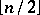denotes the largest integer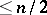.

They satisfy the following recurrence: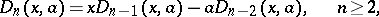where the two initial polynomials are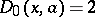and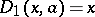. A second closed form is given byThere is a functional equation, so that ifcan be written asfor some, then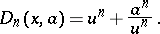The generating function is: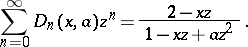Dickson polynomials satisfy a second-order differential equation which corresponds to the well-known differential equation for the classical Chebyshev polynomials. In particular, the polynomialsatisfiesDickson polynomialsare not unrelated to a well-studied class of polynomials. Consider the classical Chebyshev polynomials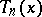, defined for each integer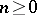by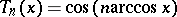. Over the complex numbers, if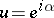so that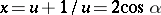,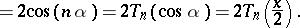Hence Dickson polynomials are related to the classical Chebyshev polynomials, and in fact some authors use the latter terminology.

L.E. Dickson was the first to seriously study various algebraic and number-theoretic properties of these polynomials. In particular, he studied these polynomials as part of his Ph.D. thesis at the University of Chicago in 1896; see also [a4]. In 1923, I. Schur [a16] suggested that these polynomials be named in honour of Dickson.

Properties and applications.

Dickson polynomials have various properties and applications in a variety of areas. Rather than trying to provide too many details here, only a few of these properties and applications are given below; see the various references for additional details. See [a10] for a survey of algebraic and number-theoretic properties as well as applications of Dickson polynomials; see also [a9] for a brief discussion of some of these properties.

Dickson polynomials play a fundamental role in the theory of permutation polynomials over finite fields. Fora prime power, let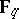denote the finite field containingelements. A polynomial with coefficients inis a permutation polynomial if it induces a one-to-one mapping on the field. It is known from [a11], p. 356, that for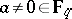, the Dickson polynomialis a permutation polynomial onif and only if the greatest common divisor ofandequals: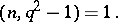(When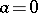,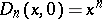induces a permutation onif and only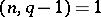.) S.D. Cohen [a3] has shown that ifis a large prime number compared to the degree of a polynomial, then the permutation polynomialessentially comes from a Dickson polynomial. See [a14] for a discussion of Dickson's work related to permutations (which Dickson called substitution quantics) and his work related to linear groups.

The real importance of Dickson polynomials in the theory of permutations comes from the Schur conjecture. Ifis an odd prime number, reduce an integral polynomialmodulo, i.e. reduce the integer coefficients modulo the prime number, so that one may viewas a function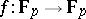, where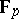denotes the field of integers modulo. Using the Dirichlet theorem on prime numbers in an arithmetic progression, it is easy to see that the polynomial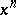permutes the fieldfor infinitely many prime numbersif and only if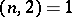. Similarly, for a non-zero integer, the Dickson polynomial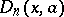permutes the fieldfor infinitely many prime numbersif and only if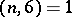, see [a10], p. 75. In 1923, I. Schur conjectured in [a16] that there are no other integral polynomials that permute the fieldfor infinitely many prime numbersother than compositions of power polynomials, Dickson polynomials, and linear polynomials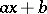(here,andmay be rational numbers). The first proof of this conjecture was given by M. Fried [a5]. More recently, in [a17] G. Turnwald proved this using only elementary (i.e., without complex analysis) arguments but the proof is still not easy. No truly easy proof is known (1998). In [a13] G.L. Mullen proved a matrix analogue of Schur's conjecture.

Given a polynomialover, define the value set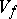ofby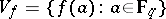. Since a polynomial of degreeover a field can have at mostroots, one has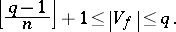Polynomials which achieve the above lower bound are called minimal value-set polynomials, see [a12]. As noted in [a7], polynomials of degreewith value sets of small cardinality (less than twice the minimum) come from Dickson polynomials. As indicated in [a2], Dickson polynomials provide one of the few classes of polynomials over finite fields whose value sets have been determined.

If the polynomials (one of each degree) of a class, called a permutable chain of polynomials, over an integral domain commute under composition, then this class must essentially come from the two classes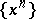and, see [a10], p. 13. Dickson polynomials provide a class of polynomials, one of each degree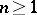, which can be solved by radicals; see [a18].

The construction of irreducible polynomials over finite fields (cf. also Irreducible polynomial) is an important problem and in [a6] it is shown how Dickson polynomials can be effectively used in this regard. For computational work involving finite fields it is useful to have various kinds of bases for extension fields. In this regard, Dickson polynomials again play important roles; see [a6] for polynomial bases and [a1] and [a15] for completely normal bases. Connections between optimal normal bases and Dickson polynomials are discussed in [a10], Sect. 7.5.

Various applications of Dickson polynomials are discussed in [a10], Chap. 7. These applications include cryptology for the secure transmission of information, pseudo-primality testing (cf. also Pseudo-prime; Probabilistic primality test), complete mappings useful in the construction of sets of mutually orthogonal Latin squares (cf. also Latin square), and character sums related to Dickson polynomials (cf. also Weyl sum).

A combinatorial application of Dickson polynomials is developed in [a8], where a study is made of Dickson–Stirling numbers. These generalize the well-studied Stirling numbers of the first and second kinds (cf. Combinatorial analysis). See [a10], Sect. 7.7, for a construction of complete sets of mutually orthogonal Latin squares of prime power orders using Dickson polynomials.

As with the classical Chebyshev polynomials, Dickson polynomials of the second kind have also been studied. These polynomials of degreewith parametermay be defined by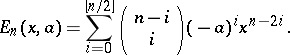Dickson polynomials of the second kind satisfy the same recurrence as those of the first kind, except that the initial polynomial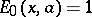. See [a10], Chap. 2, for other basic properties of these polynomials.

Contrary to the many known properties of the Dickson polynomialsof the first kind, as indicated in [a10], very few properties of Dickson polynomials of the second kind are known (1998); see, for example, [a10], p. 76, as this comment relates to permutations.

Dickson polynomials in several variables have been considered by a number of authors. For a brief survey see [a10], Sects. 2.4, 3.5, and 4.3. A connection of Dickson polynomials in several variables to circulant determinants is given in [a10], Sect. 7.8.

Dickson polynomials have been studied over a number of algebraic settings in addition to finite fields. In particular, various properties of Dickson polynomials have been obtained for the ringof integers moduloas well as for the Galois ring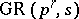(a degreeGalois extension of), infinite algebraic extensions of finite fields, and matrix rings, see [a10], Chaps. 4; 5.

How to Cite This Entry:
Dickson polynomial. Encyclopedia of Mathematics. URL: http://encyclopediaofmath.org/index.php?title=Dickson_polynomial&oldid=16913
This article was adapted from an original article by Gary L. Mullen (originator), which appeared in Encyclopedia of Mathematics - ISBN 1402006098. See original article